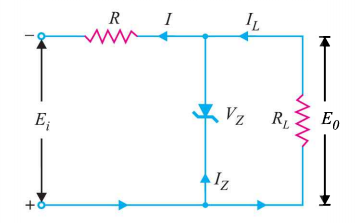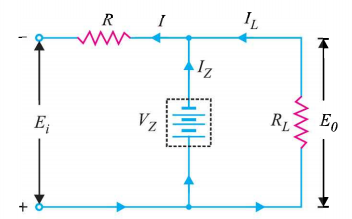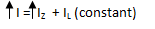# Zener Diode as Voltage Regulator

## Zener Diode as Voltage Regulator

A zener diode can be used as a voltage regulator or voltage stabilizer, to provide a constant voltage from a source whose voltage may vary over a particular range.Fig.1

The circuit connection is shown in Fig.1 .

The zener diode of zener voltage  VZ is connected reversely across the load resistance  RL across which constant output voltage EO is required.

The series resistance R is used to absorb the output voltage fluctuations, so as to maintain constant output voltage across RL .

When the circuit is properly designed, the output voltage EO remains constant even though the input voltage  Ei and load resistance Rmay vary over a wide range.

#### Case 1: Ei Variable  and RL constantFig.2

Suppose the input voltage Ei increases, since the zener is in the breakdown region, the zener diode is equivalent to a battery of voltage VZ  as shown in Fig.2 and the output voltage remains constant at VZ ( EO =VZ).

The excess voltage is dropped across R. This will cause an increase in the value of total current I. The zener will conduct the increase of current in I, while the load current remains constant.

Hence the output voltage remains constant irrespective of the change in input voltage Ei

#### Summary(Case 1)

Suppose  Ei  increases

Since zener is in breakdown region, VZ remains constant

EO = VZ

So  EO   remains constant

Excess voltage drops across R

So I increases, since   IL remains constant, IZ increasesSo the excess current is conducted by the zener diode and EO remains constant irrespective of the change in Ei

#### Case 2: Ei Constant  and RL Vaiable

Now suppose the input voltage Ei is constant but RL decreases

Since the zener diode is in breakdown region , voltage across it will remain constant at   VZ.

As the output voltage EO is equal to the zener  voltage, So EO will also remain constant at VZ.

When RL  decreases ,in order  to maintain EO constant, current through the load resistance  I will increase.

Since Ei is constant, total current  I is also constant. So the increase in load current IL will come from a decrease in zener current IZ  .Voltage drop across R =   Ei   – EO

Current through R , I = IZ + IL